### LEFT Function Examples – Excel, VBA, & Google Sheets

This tutorial demonstrates how to use the Excel LEFT Function in Excel to return the first n number of characters in a cell.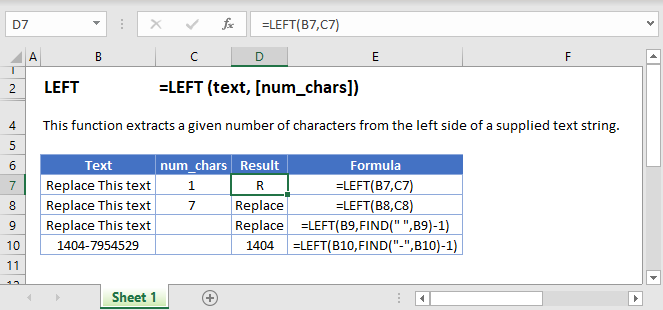## LEFT Function Overview

The LEFT Function Returns a specified number of characters from the start of a cell.

To use the LEFT Excel Worksheet Function, select a cell and type: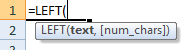(Notice how the formula inputs appear)

### LEFT function Syntax and inputs:

text – A string of text.

num_chars – The number of characters from the begining of the cell to return.

AutoMacro - VBA Code Generator

## How to use the LEFT Function in Excel:

The LEFT function extracts a specific number of characters you specify from the left (start of the string).The above extracts 7 characters from the left or the start of the string.

The second argument is optional and the default value of it is 1. Hence, it extracts 1 character from the left and gives you “R” if you omit it.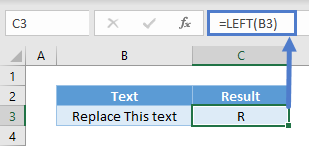### LEFT with FIND/SEARCH

In many scenarios, the number of characters we want to extract from the left varies just like first names. In this case here, we can use FIND to check which character position the space starts and minus one to exclude the space itself.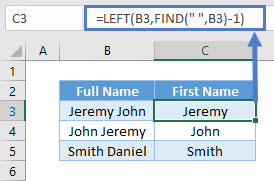You can also use SEARCH. The difference between FIND and SEARCH is that the former is case-sensitive. Finding a symbol such as space wouldn’t matter.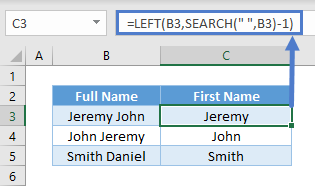### LEFT with LEN

There could be instances you know how many characters you don’t want, but not how many you want. In the eg below, there are always 4 numbers at the end. But the number of alphabets differ.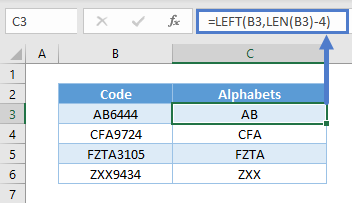LEN helps us obtain the total number of characters in the cell. And since we know we don’t want the 4 numbers at the end, simply use total length minus 4 characters to obtain the number of alphabet characters.

### LEFT with Number/Dates

Do note that LEFT is a text function. Upon using LEFT, the result is a text. For instance, you won’t be able to sum up these numbers in cell E3 after using LEFT.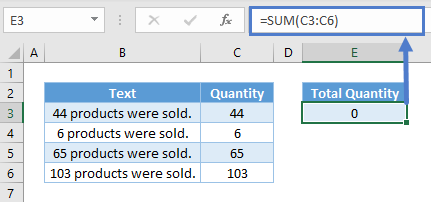The same thing happens for dates as they are recognized as serial numbers and not text. You may not need to sum dates, but it doesn’t work well in filters and PivotTables.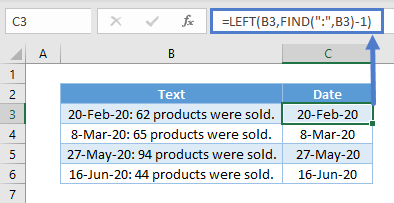To overcome the issues above, you can use VALUE to convert from text to values.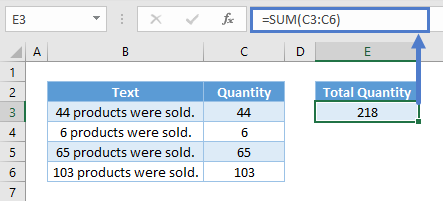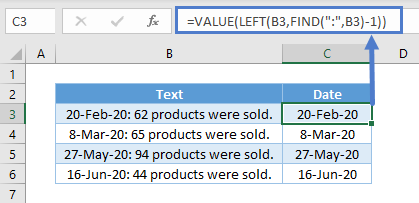## Extract number from the Left of a string

When you working with excel you need to exact a number from given detail. Sometime you need to exact number from Left and sometime you need to extract number from Right. So LEN function will help you to do this task by combining with few other functions.

### Extract number from the Left

We could combine LEFT, SUM, SUBSTITUTE with LEN as follows:

Which would give us the following results: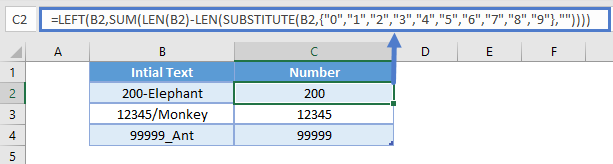### Trim text to Nth words

We could trim text to set Nth words by combining SUBSTITUTE, FIND with LEFT as follows:

Which would give us the following results in given examples.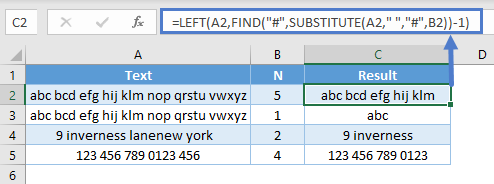### Exact initials from Name

When we collecting human information we are collecting first name and Last name separately. But when we presenting sometime we need present name with initials. In below example we will help you to provide solution to exact initials from name and also this could support to overcome from all lowercase and uppercase as well. To get this output We could combine CONCATENATE, UPPER,PROPER with LEFT as follows:

Which would give us the following results.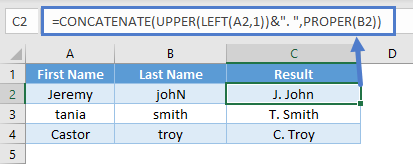### Create Email address using First name and Last name

In this example we could explain how to create EMAIL address using given first name and Last name. We could create email address by combining  CONCATENATE, LOWER with LEFT as follows: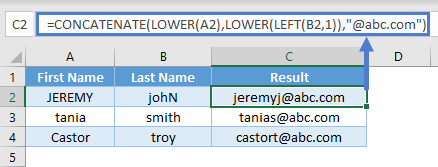## LEFT in Google Sheets

The LEFT Function works exactly the same in Google Sheets as in Excel: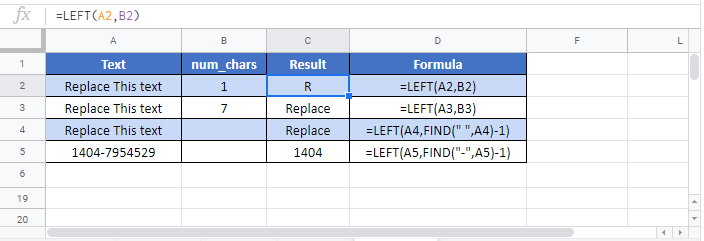## Additional Notes

If num_chars is greater than the length of the text, the LEFT Function returns all text.

## LEFT Examples in VBA

You can also use the LEFT function in VBA. Type:
`application.worksheetfunction.left(text,num_chars)`
For the function arguments (text, etc.), you can either enter them directly into the function, or define variables to use instead.

Return to the List of all Functions in Excel

## Excel Practice Worksheet

Practice Excel functions and formulas with our 100% free practice worksheets!

• Automatically Graded Exercises
• Learn Excel, Inside Excel!